Courses

# Design of Power Transmission System - MCQ Test 2

## 30 Questions MCQ Test GATE Mechanical (ME) 2022 Mock Test Series | Design of Power Transmission System - MCQ Test 2

Description
This mock test of Design of Power Transmission System - MCQ Test 2 for Mechanical Engineering helps you for every Mechanical Engineering entrance exam. This contains 30 Multiple Choice Questions for Mechanical Engineering Design of Power Transmission System - MCQ Test 2 (mcq) to study with solutions a complete question bank. The solved questions answers in this Design of Power Transmission System - MCQ Test 2 quiz give you a good mix of easy questions and tough questions. Mechanical Engineering students definitely take this Design of Power Transmission System - MCQ Test 2 exercise for a better result in the exam. You can find other Design of Power Transmission System - MCQ Test 2 extra questions, long questions & short questions for Mechanical Engineering on EduRev as well by searching above.
QUESTION: 1

Solution:
QUESTION: 2

Solution:
QUESTION: 3

### Interference in a pair of gears is avoided, if the addendum circles of both the gears intersect common tangent to the base circles within the points of tangency.

Solution:
QUESTION: 4

A spur gear has a module of 3 mm, number of teeth 16, a face width of 36 mm and a pressure angle of 20°. It is transmitting a power of 3 kW at 20 rev/s. Taking a velocity factor of 1.5, and a form factor of 0.3, the stress in the gear tooth is about

Solution:
QUESTION: 5

Given that the tooth geometry factor is 0.32 and the combined effect of dynamic load and allied factors intensifying the stress is 1.5; the minimum allowable stress (in MPa) for the gear material is

Solution:
QUESTION: 6

The resultant force on the contacting gear tooth in N is:

Solution:
QUESTION: 7

A compacting machine shown in the figure below is used to create a desired thrust force by using a rack and pinion arrangement. The input gear is mounted on tile motor shaft. The gears have involute teeth of 2 mm module.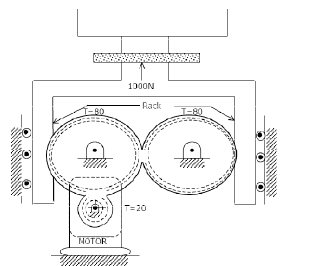If the drive efficiency is 80%, then torque required on the input shaft to create 1000 N output thrust is

Solution: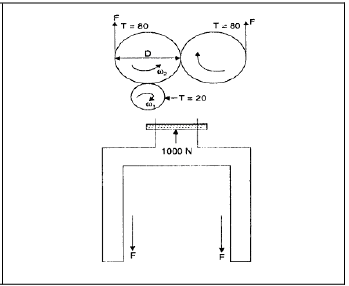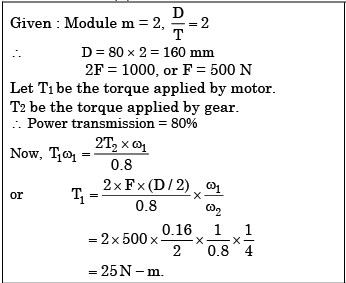QUESTION: 8

The arm OA of an epicyclic gear train shown in figure revolves counter clockwise about O with an angular velocity of 4 rad/s. Both gears are of same size. Tire angular velocity of gear C, if the sun gear B is fixed, is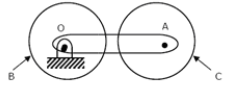Solution: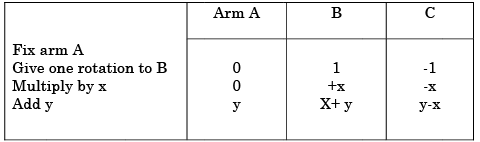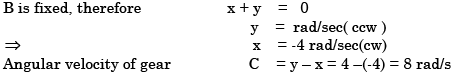QUESTION: 9

Two mating spur gears have 40 and 120 teeth respectively. The pinion rotates at 1200 rpm and transmits a torque of 20 Nm. The torque transmitted by the gear is

Solution: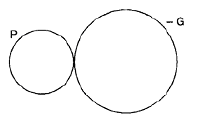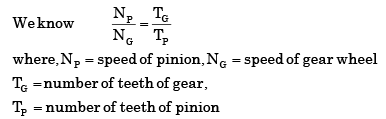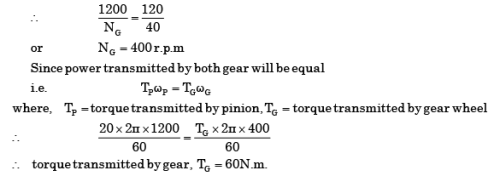QUESTION: 10

A 1.5 kW motor is running at 1440 rev/min. It is to be connected to a stirrer running at 36 rev /min. The gearing arrangement suitable for this application is

Solution:

speed reduction = 1440/36 = 40

QUESTION: 11

The velocity ratio between pinion and gear in a gear drive is 2.3, the module of teeth is 2.0 mm and sum of number of teeth on pinion and gear is 99. What is the centre distance between pinion and the gear?

Solution: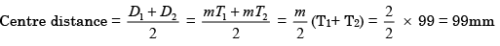QUESTION: 12

The working surface above the pitch surface of the gear tooth is termed as

Solution:
QUESTION: 13

Assertion (A ):  When one body drives another by direct contact, their contact points must have equal components of velocity normal to the surfaces at the point of contact.

Reason (R): Two  points in the same body must have the same component of velocity relative to the third body, in the direction of the line joining the two points.

Solution:
QUESTION: 14

Match List-l (Type of Gears) with List-II (Characteristics) and select the correct answer using the code given below the Lists: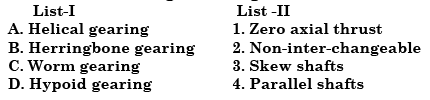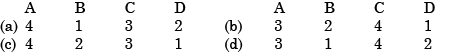Solution:
QUESTION: 15

Which type of gear is used for shaft axes having an offset?

Solution:
QUESTION: 16

When two shafts are neither parallel nor intersecting, power can be transmitted by using

Solution:
QUESTION: 17

In a single reduction, a large velocity ratio is required. The best transmission is

Solution:
QUESTION: 18

Mitre gears

Solution:
QUESTION: 19

Gearing contact is which one of the following?

Solution:

When pair of teeth touch at the pitch point ,they have for the instant pure rolling action. At any other position they have the slidingaction.

QUESTION: 20

What is the value of pressure angle generally used for involute gears?

Solution:
QUESTION: 21

An external gear with 60 teeth meshes with a pinion of 20 teeth, module being 6 mm. What is the centre distance in mm?

Solution: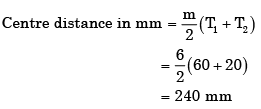QUESTION: 22

Assertion (A ): An involute rack with 20° pressure angle meshes with a pinion of 14.5° pressure angle.         Reason (R): Such  a matching is impossible.

Solution:
QUESTION: 23

Which of the following statements are correct?

1. For constant velocity ratio transmission between two gears, the common normal at the point of contact must always pass through a fixed point on the line joining the centres of rotation of the gears.

2. For involute gears the pressure angle changes with change in centre distance between gears.

3. The velocity ratio of compound gear train depends upon the number of teeth of the input and output gears only.

4. Epicyclic gear trains involve rotation of at least one gear axis about some other gear axis.

Solution:
QUESTION: 24

In involute gears the pressure angle is

Solution:

The pressure angle is always constant in involute gears.

QUESTION: 25

A certain minimum number of teeth is to be kept for a gear wheel

Solution:
QUESTION: 26

Assertion (A ): When a pair of spur gears of the same material is in mesh, the design is based on pinion.

Reason (R): For a  pair of gears of the same material in mesh, the 'strength factor' of the pinion is less than that of the gear.

Solution:
QUESTION: 27

A thin circular disc is rolling with a uniform linear speed, along a straight path on a plane surface.

Consider the following statements in this regard:

1. All points on the disc have the same velocity.

2. The centre of the disc has zero acceleration.

3. The centre of the disc has centrifugal acceleration.

4. The point on the disc making contact with the plane surface has zero acceleration of these statements

Solution:
QUESTION: 28

Consider the following statements:

1. A stub tooth has a working depth larger than that of a full-depth tooth.

2. The path of contact for involute gears is an arc of a circle.

Which of the statements given above is/are correct?

Solution:

1. A stub tooth has a working depth lower than that of a full-depth tooth.

2. The path of contact for involute gears is a line.

QUESTION: 29

Which one of the following is correct?

When two teeth profiles of gears are conjugate, the sliding velocity between them

Solution:
QUESTION: 30

Common contact ratio of a pair of spur pinion and gear is

Solution:

The ratio of the length of arc of contact to the circular pitch is known as contact ratio i.e. number of pairs of teeth in contact. The contact ratio for gears is greater than one. Contact ratio should be at least 1.25. For maximum smoothness and quietness, the contact ratio should be between 1.50 and 2.00. High-speed applications should be designed with a face-contact ratio of 2.00 or higher for best results.